American Journal of Optics and Photonics
Volume 3, Issue 1, February 2015, Pages: 17-23

Population Inversion and X-Ray Laser Gain by Electron Impact Excitation of Ni-Like Tin

Mahmoud Ahmad1, 2, Ahmed Abou El-Maaref1, *, Essam Abdel-Wahab1, Sami Allam3

1Physics Department, Faculty of Science, Al-Azhar University, Assiut, Egypt

2Physics Department, College of Science, Majmaah University, Al-Zulfi, Kingdom of Saudi Arabia

3Laboratory of Lasers and New Materials, Physics Department, Faculty of Science, Cairo University, Giza, Egypt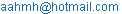(A. A. El-Maaref)

Mahmoud Ahmad, Ahmed Abou El-Maaref, Essam Abdel-Wahab, Sami Allam. Population Inversion and X-Ray Laser Gain by Electron Impact Excitation of Ni-Like Tin. American Journal of Optics and Photonics.Vol.3, No. 1, 2015, pp. 17-23. doi: 10.11648/j.ajop.20150301.14

Abstract: Excitation energies and transition probabilities for the 1s22s22p63s23p63d10, and the 1s22s22p63s23p63d94l (l= s, p, d, and f) configurations in nickel-like tin have been used to calculate the reduced population of  99 fine structure levels over a wide range of electron densities and at different electron plasma temperatures. The gain coefficients for those transitions with positive population inversion factors have been calculated as a function of electron density. This study is concerned with the transitions which produce positive gain at XUV and X-ray spectral regions.

Keywords: Ni-Like Sn, Population Inversion, Laser Gain, X-Ray, XUV

Contents

1. Introduction

Since the first demonstration of X-ray laser gain in neon-like selenium , the electron-collisional excitation scheme has proven to be the most successful method on the route towards high output power and gain. The nickel-like ground state (3s2 3p6 3d10 1S0) is in a similarity with the ground state of neon isoelectronic sequence (1s2 2s22p6 1S0). A promising values of laser gain have been obtained of neon-like ions such as selenium, germanium, and titanium . In the last few years, transitions of the 4d-4p states with J = 0,1 in nickel-like ions at wavelengths between 7.3 and 14.7 nm have been reported [3-5]. Obtaining XUV (extreme ultraviolet) and X-ray laser transitions from nickel-like ions using different methods have been discussed in previous works [6-9]. The lasing at wavelength of 3.6 nm has been demonstrated in gold . Recently, lasing in many nickel-like lanthanide ions has been demonstrated by Daido et al. , and Nilsen and Moreno . Lasing at wavelengths from 6 to 8 nm was obtained with a drive energy as low as 200 J . The use of low-Z nickel-like ions as a candidate for a tabletop system has been proposed, and preliminary gain observation at 20.4 nm in nickel-like niobium has been reported .  It is clear that the demonstration of gain in low-Z nickel-like ions may lead to significant progress in developing a small scale system operating at short wavelengths. Nickel-like X-ray lasing in tin is in fact the first proposed nickel-like ion laser, and gain production in an imploding gas puffer was discussed in 1985 . Experimentally, it has been previously investigated using an exploding foil target , in which an indication of amplification on one of the J=0,1 for the 4d-4p transitions at 11.91 nm was reported. Two techniques for gain demonstration were used. One is the prepulse technique, which has been successful in producing low-Z neon-like lasers [15-19]. The other technique is the use of curved targets, which has been proven as an effective method to enhance the J=0 to J=1 laser in neon-like germanium [20,21] and in nickel-like lanthanide ions [11,12]. With these techniques, X-ray lasing in nickel-like tin with gain length of 4.8 (dimensionless) has been observed [22-23]. The purpose of this work is the using of available atomic data to calculate the reduced populations and gain coefficients for nickel-like tin over a wide range of electron density at different electron temperatures to examine the possibility of obtaining XUV and X-ray laser emissions.

2. Computation of Gain Coefficient

The possibility of producing X-ray laser emissions from nickel-like tin via electron-collisional pumping is computed at different plasma temperatures and electron densities. The reduced population densities can be calculated using the quasi-steady state (QSS) approximation by solving the coupled rate equations [24-26]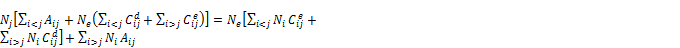(1)

where Nj is the population of the level j, Aji is the spontaneous decay rate from the upper level j to the lower level i,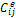is the electron collisional excitation rate coefficient, and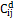is the electron collisional de-excitation rate coefficient, which is related to electron collisional excitation rate coefficient by the relation [27,28].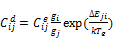(2)

where gi and gj are the statistical weights of the lower and the upper levels, respectively. The electron impact excitation rates are expressed via effective collision strengths by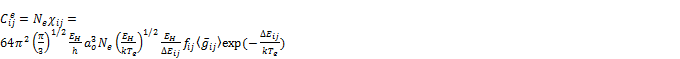(3)

where Ne is the electron density, χij is collisional excitation rate coefficient, h is the Plank’s constant, ao is the radius of the first Bohr orbit, EH is the ionization potential of the hydrogen ground state (13.6 eV), k is the Boltzman constant, Te is the electron temperature, ΔEij is the energy gap between levels i and j, fij is the oscillator strengths of the transition i - j, and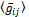is the Maxwellian-averaged effective Gaunt factor. The Gaunt factor is used as a multiplicative correction to emission results when the calculations are performed using classical physics techniques , it fitted to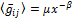with μ=0.17 and β=0.47 for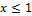, where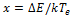. For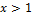a constant of 0.17 is assumed. While μ=0.63 and β=0.15 for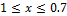. These parameters have been selected to simulate plasmas which can be produced by presently available technologies . The values of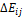and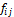have been taken from ref. . The actual population density NJ of the jth level is obtained from the identity :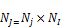(4)

NI is the quantity of ions which reach to ionization stage I, which given by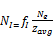(5)

here fI is the fractional abundance of nickel-like ionization stage, Ne is the electron density, and avg is the average degree of ionization [31,32]. Since the populations calculated from equation (1) are normalized,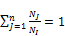(6)

where n is the number of the levels under consideration, the quantity NJ/NI obtained from Eq. (1) is the fractional population, then the reduced fractional population  Nj/gj and Ni/gi can be calculated . After the ion is collisonally pumped, the produced plasma will transfer the pumped quanta to other levels, as a result of this process, the population inversions between the upper and lower levels will be obtained. Once a population inversion has been ensured, a positive gain through F>0  is obtained by the relation,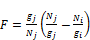(7)

where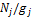and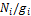are the reduced populations of the upper level and lower levels, respectively. Equation (7) has been used to calculate the gain coefficient (α) for Doppler broadening of the various transitions in the Sn22+ ion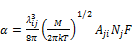(8)

M denotes ion mass, λij is the transition wavelength in cm, T is the ion temperature in K, and j, i represent the upper and lower transition levels, respectively. As seen in equation (8) the gain coefficient is expressed in terms of the upper state density (Nj). This quantity Nj depends on how the upper state is populated, as well as on the density of the initial source state.

3. Results and Discussions

3.1. Level Population

The reduced population densities have been calculated for 99 fine structure levels arising from 1s22s22p63s23p63d10 and 1s22s22p63s23p63d94l (l= s, p, d, and f) configurations belonging to nickel-like Sn which emit radiation in the XUV and soft X-ray spectral regions. The calculation was performed by solving the coupled rate equations (Eq.1) simultaneously. Our calculations of reduced populations as a function of electron densities are shown in figures (1-6) at different plasma temperatures. In the present calculations, the spontaneous radiative decay rate and electron collisional processes between all levels under consideration have been taken into account. The behavior of level populations of Sn22+ can be explained as follows: in general, at low electron densities the reduced population density is proportional to electron density, where the excitation to an excited state is followed immediately by radiation decay, and the collisional mixing of excited levels can be ignored. The mixing coefficients of some excited levels can take the forms:

3d94d(1S) = 0.99432 3d94d(1S)+0.10637 3d94d(3P)

3d94d(3G) = 1.000 3d94d(3G)

where the numbers 0.99432, 0.10637, and 1.000 are the configuration mixing coefficients. The mixing coefficients less than 0.1 are omit.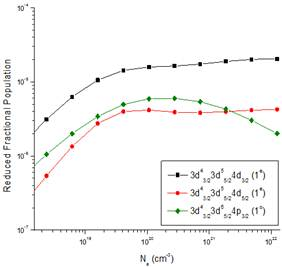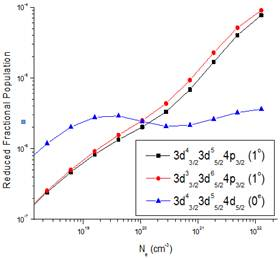Fig. 1. Reduced fractional population for selected levels of Ni-like tin at electron temperature 100 eV.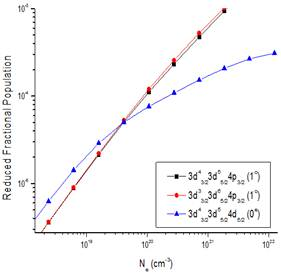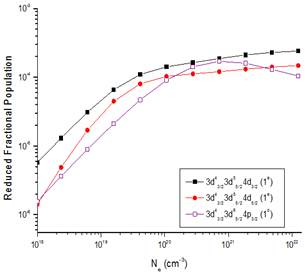Fig. 2. Reduced fractional population for selected levels of Ni-like tin at electron temperature 200 eV.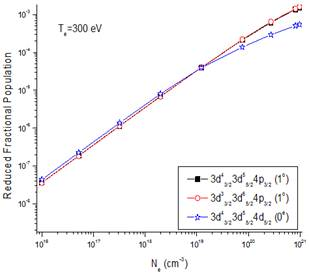Fig. 3. Reduced fractional population for selected levels of Ni-like tin at electron temperatures 300 and 400 eV.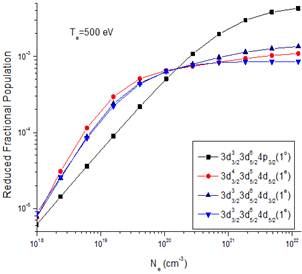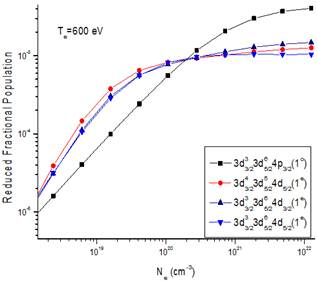Fig. 4. Reduced fractional population for selected levels of Ni-like tin at electron temperatures 500, 600 eV.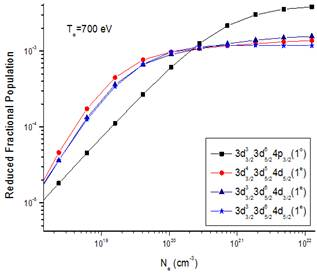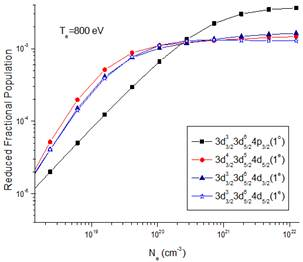Fig. 5. Reduced fractional population for selected levels of Ni-like tin at electron temperatures 700, 800 eV.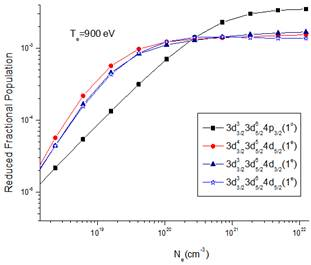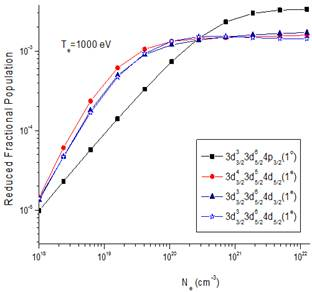Fig. 6. Reduced fractional population for selected levels of Ni-like tin at electron temperatures 900, 1000 eV.

At high electron densities the radiative decay to all levels will be negligible compared to collisional depopulations and all level populations become independent of electron density and are approximately equal. As shown in figures (1-6), the relative sublevel populations grow with the electron density and the temperature due to the increase of collisional excitation rates. For a given temperature, in the region of low electron density,  the populations of the configuration array 3d94p are smaller  than those of the 3d94d levels, this behavior   is due to their strong  radiative  decay; however, in the region of high  electron density, the populations become distributed according to Boltzmann distribution because the  collisional de-excitation rates exceed the radiative transition rates . The configuration array 3d94d upper laser levels are more populated than the 3d94p lower laser levels in electron collisional excitation. In spite of the fact that the 3d94d upper laser levels are optically forbidden and have no optical connection to the ground level, and it is strongly optically connected to the 3d94p levels therefore laser transition is favorable . The population inversion is largest where electron collisional de-excitation rate for upper level is comparable to the radiative decay for this level [18,24]. The positive laser gain is only obtained when the inversion factor F is greater than zero. In order to work in the XUV and X-ray spectral regions, transitions between any two levels producing photons with wavelengths between 3 and 100 nm have been selected. The electron density at which the population reaches corona equilibrium is approximately equals to A/D, where A is the radiative decay rate and D is the collisional de-excitation rate . Refs. [2,33] reported results of reduced populations and laser gain of Ni-like Au and Eu. Just for estimating our result accuracies we will compare the present calculations of some computed parameters such as reduced populations and gain with those in refs. [2,33]. For example, the population inversion for the transitions 3d94p (0) - 3d94d (1) are about 6×10-3  and 2×10-3  at 1000 eV. In the present work the population inversion of the transition 3d94p (1) - 3d94d (1) is about 0.5×10-3 at 1000 eV. It is clear that the present results are in the same order of the previous results [2,33] but if we want to do a precise comparisons it should be between the same ions in the same conditions, which are not available for the ion under consideration.

3.2. Gain Coefficient

As a result of population inversion there will be a positive gain in the laser medium. Equation (8) has been used to calculate the gain coefficient for the Doppler broadening of various transitions in Sn22+. The present calculations of gain values as a function of electron densities at different electron temperatures are shown in figures (7,8). The figures show that the population inversions occur for several transitions in Sn22+, however, the largest gain has been existed for the (3d43/23d55/24d5/2)0 - (3d43/23d55/24p3/2)1 transition producing gain value of 74.46 cm-1 with wavelength of 11.1 nm and electron temperature 300 eV, and for the transition (3d43/23d55/24d5/2)0 -  (3d33/23d65/24p3/2)1 which producing gain of 15.06 cm-1 with wavelength of 11.94 nm and Te=300 eV. A summary  of the most promising X-ray laser gain from nickel-like tin have been listed in table 1. For electron densities and electron temperatures that are typical of laboratory high density plasma sources, such as laser produced plasmas, it is possible to create a quasi-stationary population inversion between the 3d94d and 3d94p states with J=0,1 in Sn22+. There is a trend of the gain factor with the electron temperature, where the gain factor decreases by increasing the electron temperature, such as shown in Fig. 7, while in Fig. 8 the gain increases by increasing electron temperature. Indirectly, the gain factor depends on the collision strengths, where the collision strengths of the transitions 3d43/23d55/24d5/2 (0e)- 3d43/23d55/24p3/2 (1o) and 3d43/23d55/24d5/2 (0e)- 3d33/23d65/24p3/2 (1o) decrease by increasing the electron temperature (Fig. 7), while the collision strengths of the transition 3d33/23d65/24d3/2 (1e)- 3d33/23d65/24p3/2 (1o) increase by  increasing the electron temperature (Fig. 8) . Our calculations show that, under a favorable conditions, a significant laser gain for these transitions in the XUV and soft X-ray spectral regions can be achieved in nickel-like Sn.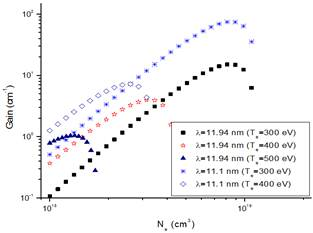Fig. 7. The calculated gain for different transitions emitted wavelengths of 11.94 and 11.1 nm at different electron temperatures.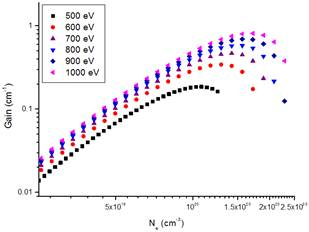Fig. 8. The calculated gain for the transition with wavelength, λ = 17.2 nm at different electron temperatures.

Table 1. The largest gain values of obtained X-ray laser emissions from Ni-like Sn ion.

 Upper level Lower level Ne (cm-3) λ (nm) Te (eV) αmax 3s23p21/23p43/23d43/23d55/24d5/2 (0e) 3s23p21/23p43/23d43/23d55/24p3/2 (1o) 8.140×1018 11.1 300 74.46 3s23p21/23p43/23d43/23d55/24d5/2 (0e) 3s23p21/23p43/23d43/23d55/24p3/2 (1o) 2.594×1018 11.1 400 7.217 3s23p21/23p43/23d43/23d55/24d5/2 (0e) 3s23p21/23p43/23d33/23d65/24p3/2 (1o) 8.140×1018 11.94 300 15.06 3s23p21/23p43/23d43/23d55/24d5/2 (0e) 3s23p21/23p43/23d33/23d65/24p3/2 (1o) 3.138×1018 11.94 400 3.948 3s23p21/23p43/23d33/23d65/24d3/2 (1e) 3s23p21/23p43/23d33/23d65/24p3/2 (1o) 1.718×1020 17.20 1000 0.811

4. Conclusion

The analysis of the present work shows that electron collisional pumping is a suitable technique for attaining population inversion and offering the potential for laser emission in the spectral region between 3 and 100 nm from the Sn22+ ion. Only transitions with significant positive gain have been considered. This class of lasers can be achieved under the suitable conditions of pumping power as well as electron density. If the positive gains obtained previously for some transitions in the Sn22+ion together with the calculated parameters could be achieved experimentally, then a successful low cost electron collisional pumping of XUV and soft X-ray lasers can be developed for various applications.

References

1. D.L. Matthews, P.L. Hagelstein, M.D. Rosen, M.J. Eckart, N.M. Ceglio, A.U. Hazi, H. Medecki, B.J. MacGowan, J.E. Trebes, B.L. Whitten, E.M. Campbell, C.W. Hatcher, A.M. Hawryluk, R.L. Kaufman, L.D. Pleasance, G. Rambach, J.H. Scofield, G. Stone, T.A. Weaver, Phys. Rev. Lett. 54 (1985) 110.
2. Wessameldin S. Abdelaziz, Hamed Mahmoud Hamed Ibrahim, Opt. Photonics J. 1 (2011) 110.
3. J. Zhang, A.G. MacPhee, J. Nilsen, J. Lin, T.W. Barbee, Jr., C. Danson, M.H. Key, C.L.S. Lewis, D. Neely, R.M.N. O'Rourke, G.J. Pert, R. Smith, G.J. Tallents, J.S. Wark, E. Wolfrum, Phys. Rev. Lett. 78 (1997) 3856.
4. J. Zhang, A.G. MacPhee, J. Lin, E. Wolfrum, R. Smith, C. Danson, M.H. Key, C.L.S. Lewis, D. Neely, J. Nilsen, G.J. Pert, G.J. Tallents, J.S. Wark, Science 276 (1997) 1097.
5. R. Tommasini, F. Loewenthal, J.E. Balmer, Phys. Rev. A 59 (1999) 1577.
6. S. Maxon, P. Hagelstein, K. Reed, J. Scofield, J. Appl. Phys. 57 (1985) 971.
7. B.J. MacGowan, S. Maxon, P.L. Hagelstein, C.J. Keane, R.A. London, D.L. Matthews, M.D. Rosen, J.H. Scofield, D.A. Whelan, Phys. Rev. Lett. 59 (1987) 2157.
8. B.J. MacGowan, S. Maxon, C.J. Keane, R.A. London, D.L. Matthews, D.A. Whelan, J. Opt. Soc. Am. B 5 (1988) 1858.
9. B.J. MacGowan, S. Maxon, L.B. Da Silva, D.J. Fields, C.J. Keane, D.L. Matthews, A.L. Osterheld, J.H. Scofield, G.M. Shimkaveg, J.F. Stone, Phys. Rev. Lett. 65 (1990) 420.
10. B.J. MacGowan, L.B. Da Silva, D.J. Fields, C.J. Keane, K.A. Koch, R.A. London, D.L. Matthews, S. Maxon, S. Mrowka, A.L. Osterheld, J.H. Scofield, G.M. Shimkaveg, J.E. Trebes, R.S. Walling, Phys. Fluids B 4 (1992) 2326.
11. H. Daido, Y. Kato, K. Murai, S. Ninomiya, R. Kodama, G. Yuan, Y. Oshikane, M. Takagi, H. Takabe, F. Koike, Phys. Rev. Lett. 75 (1995) 1074.
12. J. Nilsen, J.C. Moreno, Opt. Lett. 20 (1995) 1386.
13. P.L. Hagelstein, in Proceedings of the OSA Meeting on Short Wavelength Coherent Radiation: Generation and Applications, edited by R.W. Facone and J. Kirz ,Optical Society of America, Washington, DC, 1988, pp 28.
14. G.D. Enright, J. Dunn, D.M. Villeneuve, S. Maxon, H.A. Baldis, A.L. Osterheld, B. La Fountaine, J. C. Kieffer, M. Nantel, and H. Pépin, X Ray Lasers 1992, edited by E.E. Fill , Institute of Physics, Bristol, 1993, pp 45.
15. Y. Li, G. Pretzler, E.E. Fill, Phys.Rev. A 52 (1995) R3433.
16. J. Nilsen, B.J. MacGowan, L.B. Da Silva, J.C. Moreno, Phys. Rev. A 48 (1993) 4682.
17. J. Nilsen, J.C. Moreno, B.J. MacGowan, J.A. Koch, Appl. Phys. B 57 (1993) 309.
18. E.E. Fill, Y. Li, D. Schlögl, J. Steingruber,  J. Nilsen, Opt. Lett. 20 (1995) 374.
19. Y. Li, G. Pretzler, E.E. Fill, Opt. Lett. 20 (1995) 1026.
20. R. Kodama, D. Neely, Y. Kato, H. Daido, K. Murai, G. Yuan, A. MacPhee, C.L. Lewis, Phys. Rev. Lett. 73 (1994) 3215.
21. H. Daido, R. Kodama, K. Murai, G. Yuan, M. Takagi, Y. Kato, I.W. Choi, C.H. Nam, Opt. Lett. 20 (1995) 61.
22. Y. Li, G. Pretzler, P. Lu, E.E. Fill, Phys.Rev A. 53 (1996) R652.
23. F. Staub, M. Braud, J.E. Balmer, J. Nilsen, S. Bajt, Phys. Rev A. 70 (2004) 013813.
24. U. Feldman, A.K. Bhatia, S. Suckewer, J. Appl. Phys. 54 (1983) 2188.
25. U. Feldman, J.F. Seely, G.A. Doschek, J. Appl. Phys. 59 (1986) 3953.
26. U. Feldman, G.A. Doschek, J.F. Seely, A.K. Bhatia, J. Appl. Phys. 58 (1985) 2909.
27. G. Chapline, L. Wood, Phys. Today 28 (1975) 40.
28. A.V. Vinogradov, V.N. Shlyaptsev, Sov. J. Quantum Electron. 10 (1980) 754.
29. L.J. Palumpo, R.C. Elton, J. Opt. Soc. Am. 67 (1977) 480.
30. M.A. Dopita and R.S. Sutherland, Astrophysics of the diffuse universe, Astronomy and astrophysics library, Springer  (2003) pp 128.
31. Y.Q. Liang, J.Y. Zhong, At. Data Nucl. Data Tables 94 (2008) 807.
32. W.H. Goldstein, J. Oreg, A. Zigler, A. Bar-Shalom, M. Klapisch, Phys.Rev. A 38 (1988) 1797.
33. Wessameldin S. Abdelaziz, Th.M. ElSherbini, Opt. Laser Technol. 42 (2010) 699.
34. I.I. Sobelman, Introduction to the theory of atomic spectra, International Seriesof Monographs in Natural Philosophy, Vol. 40, Pergamon Press, 1979.
35. A.V. Vinogradov, I.I. Sobelman, E.A. Yukov, Sov. J. Quantum Electron. 7 (1977)32 .
36. A.A. El-Maaref, M.A.M. Uosif, S.H. Allam, Th.M. El-Sherbini, Can. J. Phys. 91 (2013) 981.

 Contents 1. 2. 3. 3.1. 3.2. 4.
Article ToolsAbstractPDF(347K)